# Verilog_Overview.pdf

2. Jun 2023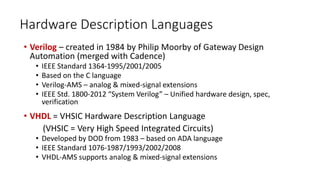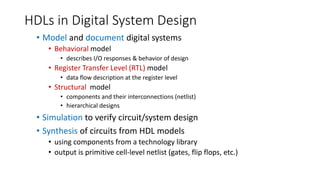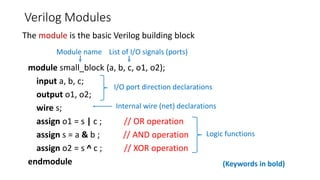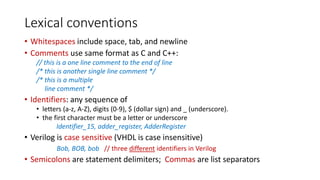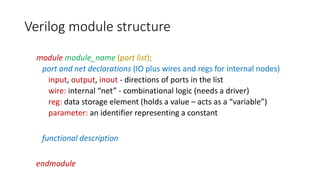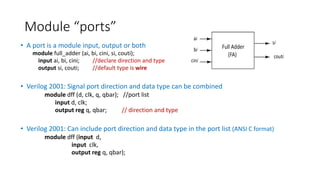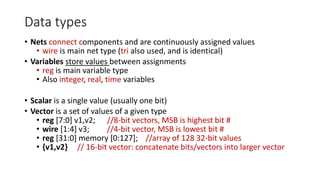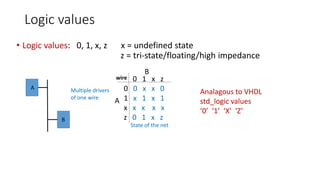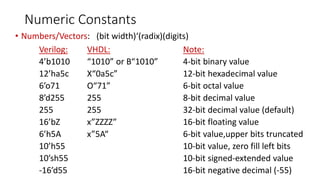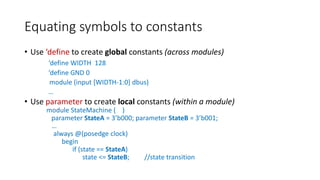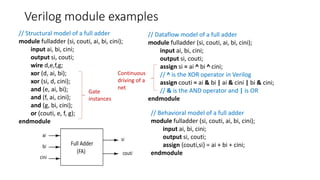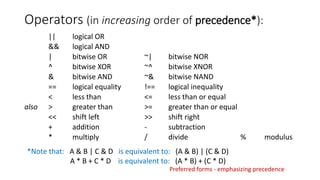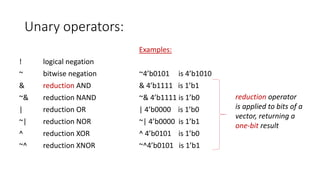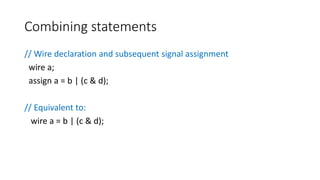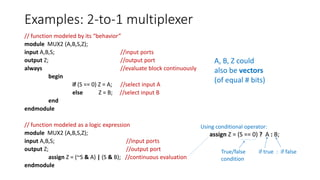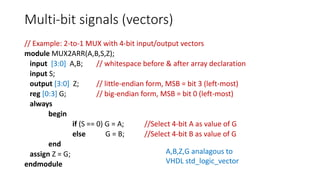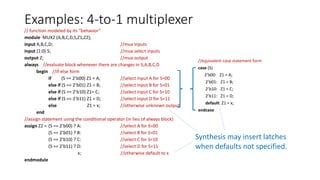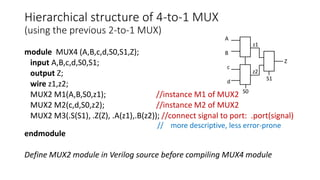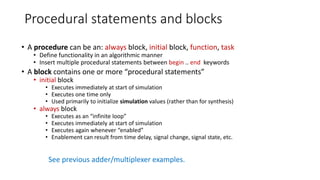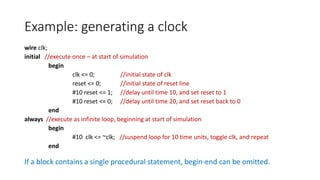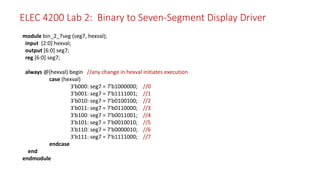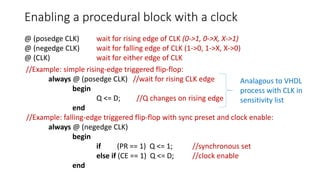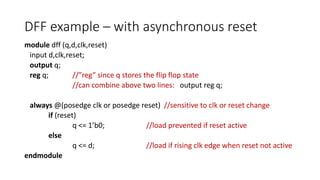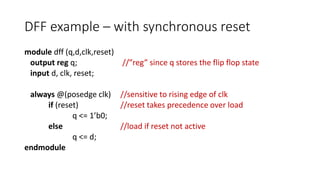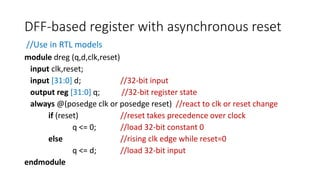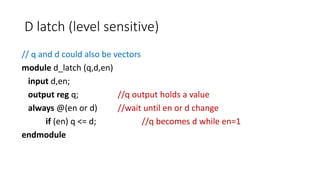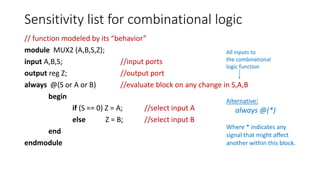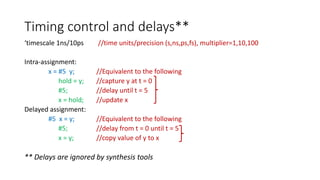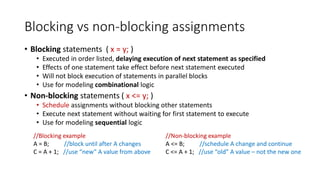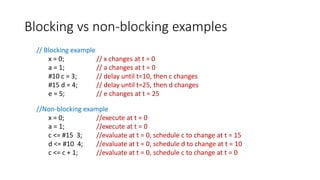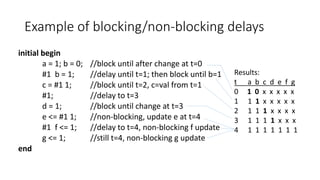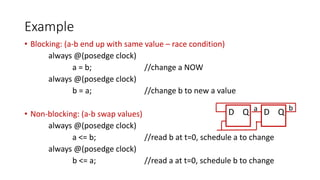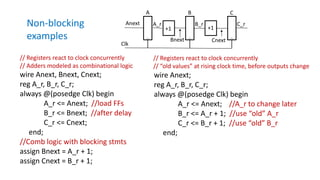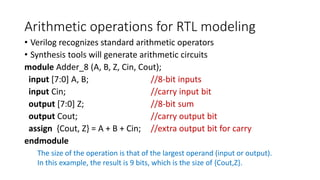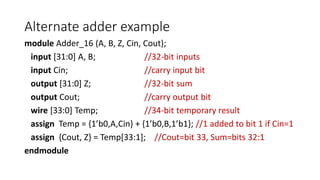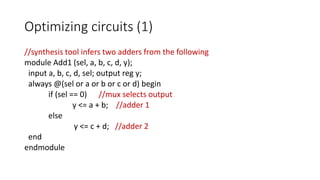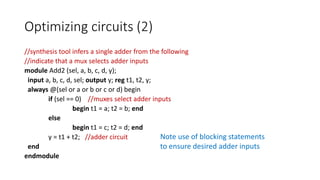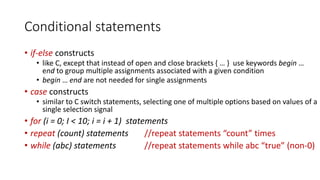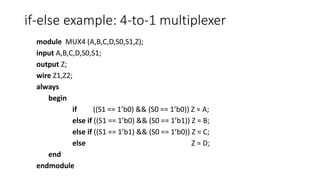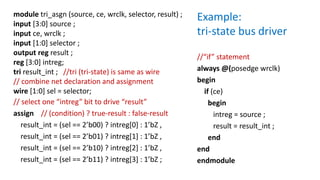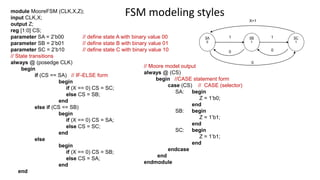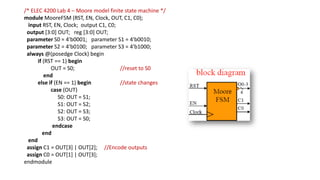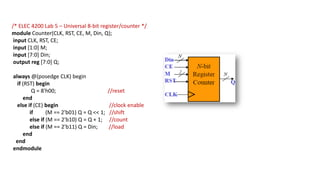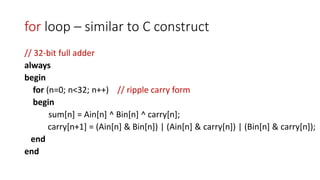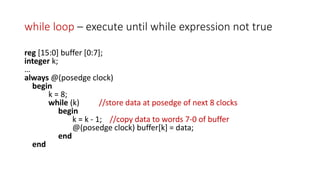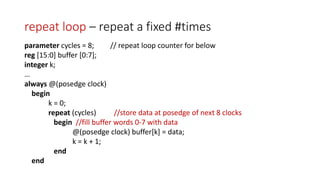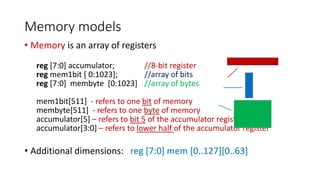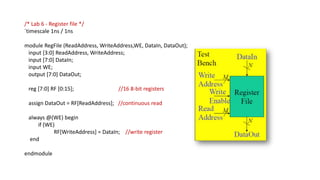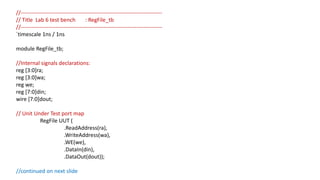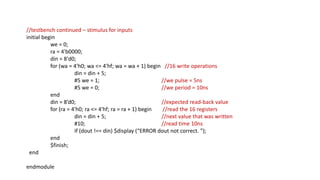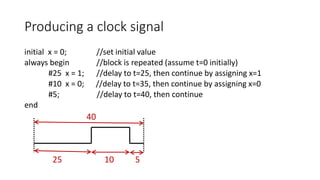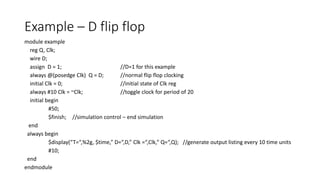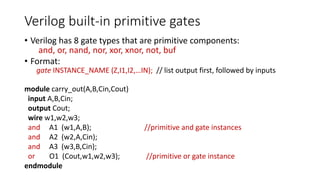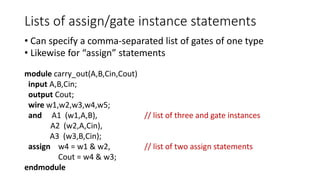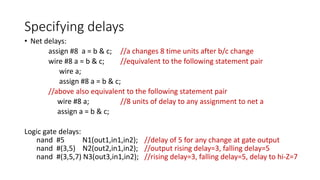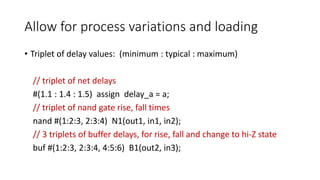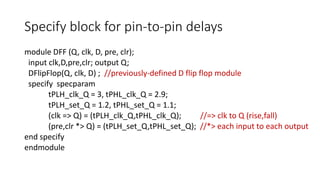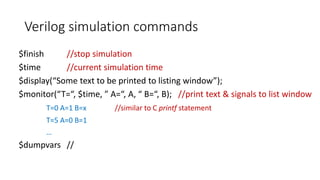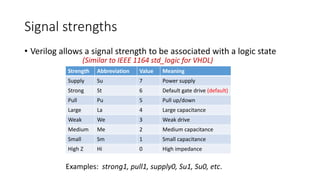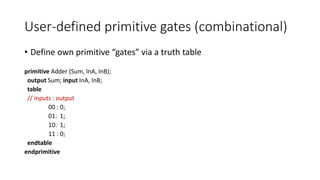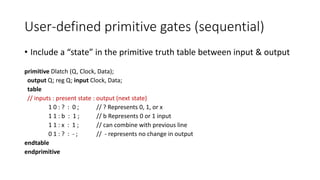1 von 62

### Verilog_Overview.pdf

• 1. Verilog modeling* for synthesis of ASIC designs * for native speakers of VHDL ELEC 4200 Victor P. Nelson
• 2. Hardware Description Languages • Verilog – created in 1984 by Philip Moorby of Gateway Design Automation (merged with Cadence) • IEEE Standard 1364-1995/2001/2005 • Based on the C language • Verilog-AMS – analog & mixed-signal extensions • IEEE Std. 1800-2012 “System Verilog” – Unified hardware design, spec, verification • VHDL = VHSIC Hardware Description Language (VHSIC = Very High Speed Integrated Circuits) • Developed by DOD from 1983 – based on ADA language • IEEE Standard 1076-1987/1993/2002/2008 • VHDL-AMS supports analog & mixed-signal extensions
• 3. HDLs in Digital System Design • Model and document digital systems • Behavioral model • describes I/O responses & behavior of design • Register Transfer Level (RTL) model • data flow description at the register level • Structural model • components and their interconnections (netlist) • hierarchical designs • Simulation to verify circuit/system design • Synthesis of circuits from HDL models • using components from a technology library • output is primitive cell-level netlist (gates, flip flops, etc.)
• 4. Verilog Modules module small_block (a, b, c, o1, o2); input a, b, c; output o1, o2; wire s; assign o1 = s | c ; // OR operation assign s = a & b ; // AND operation assign o2 = s ^ c ; // XOR operation endmodule I/O port direction declarations Logic functions The module is the basic Verilog building block Module name List of I/O signals (ports) Internal wire (net) declarations (Keywords in bold)
• 5. Lexical conventions • Whitespaces include space, tab, and newline • Comments use same format as C and C++: // this is a one line comment to the end of line /* this is another single line comment */ /* this is a multiple line comment */ • Identifiers: any sequence of • letters (a-z, A-Z), digits (0-9), \$ (dollar sign) and _ (underscore). • the first character must be a letter or underscore Identifier_15, adder_register, AdderRegister • Verilog is case sensitive (VHDL is case insensitive) Bob, BOB, bob // three different identifiers in Verilog • Semicolons are statement delimiters; Commas are list separators
• 6. Verilog module structure module module_name (port list); port and net declarations (IO plus wires and regs for internal nodes) input, output, inout - directions of ports in the list wire: internal “net” - combinational logic (needs a driver) reg: data storage element (holds a value – acts as a “variable”) parameter: an identifier representing a constant functional description endmodule
• 7. Module “ports” • A port is a module input, output or both module full_adder (ai, bi, cini, si, couti); input ai, bi, cini; //declare direction and type output si, couti; //default type is wire • Verilog 2001: Signal port direction and data type can be combined module dff (d, clk, q, qbar); //port list input d, clk; output reg q, qbar; // direction and type • Verilog 2001: Can include port direction and data type in the port list (ANSI C format) module dff (input d, input clk, output reg q, qbar);
• 8. Data types • Nets connect components and are continuously assigned values • wire is main net type (tri also used, and is identical) • Variables store values between assignments • reg is main variable type • Also integer, real, time variables • Scalar is a single value (usually one bit) • Vector is a set of values of a given type • reg [7:0] v1,v2; //8-bit vectors, MSB is highest bit # • wire [1:4] v3; //4-bit vector, MSB is lowest bit # • reg [31:0] memory [0:127]; //array of 128 32-bit values • {v1,v2} // 16-bit vector: concatenate bits/vectors into larger vector
• 9. Logic values • Logic values: 0, 1, x, z x = undefined state z = tri-state/floating/high impedance 0 1 x z 0 0 x x 0 1 x 1 x 1 x x x x x z 0 1 x z wire Multiple drivers of one wire A B A B State of the net Analagous to VHDL std_logic values ‘0’ ‘1’ ‘X’ ‘Z’
• 10. Numeric Constants • Numbers/Vectors: (bit width)‘(radix)(digits) Verilog: VHDL: Note: 4’b1010 “1010” or B“1010” 4-bit binary value 12’ha5c X“0a5c” 12-bit hexadecimal value 6’o71 O“71” 6-bit octal value 8’d255 255 8-bit decimal value 255 255 32-bit decimal value (default) 16’bZ x”ZZZZ” 16-bit floating value 6’h5A x”5A“ 6-bit value,upper bits truncated 10’h55 10-bit value, zero fill left bits 10’sh55 10-bit signed-extended value -16’d55 16-bit negative decimal (-55)
• 11. Equating symbols to constants • Use ‘define to create global constants (across modules) ‘define WIDTH 128 ‘define GND 0 module (input [WIDTH-1:0] dbus) … • Use parameter to create local constants (within a module) module StateMachine ( ) parameter StateA = 3’b000; parameter StateB = 3’b001; … always @(posedge clock) begin if (state == StateA) state <= StateB; //state transition
• 12. Verilog module examples // Structural model of a full adder module fulladder (si, couti, ai, bi, cini); input ai, bi, cini; output si, couti; wire d,e,f,g; xor (d, ai, bi); xor (si, d, cini); and (e, ai, bi); and (f, ai, cini); and (g, bi, cini); or (couti, e, f, g); endmodule // Dataflow model of a full adder module fulladder (si, couti, ai, bi, cini); input ai, bi, cini; output si, couti; assign si = ai ^ bi ^ cini; // ^ is the XOR operator in Verilog assign couti = ai & bi | ai & cini | bi & cini; // & is the AND operator and | is OR endmodule // Behavioral model of a full adder module fulladder (si, couti, ai, bi, cini); input ai, bi, cini; output si, couti; assign {couti,si} = ai + bi + cini; endmodule Gate instances Continuous driving of a net
• 13. Operators (in increasing order of precedence*): || logical OR && logical AND | bitwise OR ~| bitwise NOR ^ bitwise XOR ~^ bitwise XNOR & bitwise AND ~& bitwise NAND == logical equality !== logical inequality < less than <= less than or equal also > greater than >= greater than or equal << shift left >> shift right + addition - subtraction * multiply / divide % modulus *Note that: A & B | C & D is equivalent to: (A & B) | (C & D) A * B + C * D is equivalent to: (A * B) + (C * D) Preferred forms - emphasizing precedence
• 14. Unary operators: Examples: ! logical negation ~ bitwise negation ~4’b0101 is 4’b1010 & reduction AND & 4’b1111 is 1’b1 ~& reduction NAND ~& 4’b1111 is 1’b0 | reduction OR | 4’b0000 is 1’b0 ~| reduction NOR ~| 4’b0000 is 1’b1 ^ reduction XOR ^ 4’b0101 is 1’b0 ~^ reduction XNOR ~^4’b0101 is 1’b1 reduction operator is applied to bits of a vector, returning a one-bit result
• 15. Combining statements // Wire declaration and subsequent signal assignment wire a; assign a = b | (c & d); // Equivalent to: wire a = b | (c & d);
• 16. Examples: 2-to-1 multiplexer // function modeled by its “behavior” module MUX2 (A,B,S,Z); input A,B,S; //input ports output Z; //output port always //evaluate block continuously begin if (S == 0) Z = A; //select input A else Z = B; //select input B end endmodule // function modeled as a logic expression module MUX2 (A,B,S,Z); input A,B,S; //input ports output Z; //output port assign Z = (~S & A) | (S & B); //continuous evaluation endmodule A, B, Z could also be vectors (of equal # bits) Using conditional operator: assign Z = (S == 0) ? A : B; True/false condition if true : if false
• 17. Multi-bit signals (vectors) // Example: 2-to-1 MUX with 4-bit input/output vectors module MUX2ARR(A,B,S,Z); input [3:0] A,B; // whitespace before & after array declaration input S; output [3:0] Z; // little-endian form, MSB = bit 3 (left-most) reg [0:3] G; // big-endian form, MSB = bit 0 (left-most) always begin if (S == 0) G = A; //Select 4-bit A as value of G else G = B; //Select 4-bit B as value of G end assign Z = G; endmodule A,B,Z,G analagous to VHDL std_logic_vector
• 18. Examples: 4-to-1 multiplexer // function modeled by its “behavior” module MUX2 (A,B,C,D,S,Z1,Z2); input A,B,C,D; //mux inputs input [1:0] S; //mux select inputs output Z; //mux output always //evaluate block whenever there are changes in S,A,B,C,D begin //if-else form if (S == 2’b00) Z1 = A; //select input A for S=00 else if (S == 2’b01) Z1 = B; //select input B for S=01 else if (S == 2’b10) Z1= C; //select input C for S=10 else if (S == 2’b11) Z1 = D; //select input D for S=11 else Z1 = x; //otherwise unknown output end //assign statement using the conditional operator (in lieu of always block) assign Z2 = (S == 2’b00) ? A: //select A for S=00 (S == 2’b01) ? B: //select B for S=01 (S == 2’b10) ? C: //select C for S=10 (S == 2’b11) ? D: //select D for S=11 x; //otherwise default to x endmodule //equivalent case statement form case (S) 2’b00: Z1 = A; 2’b01: Z1 = B; 2’b10: Z1 = C; 2’b11: Z1 = D; default: Z1 = x; endcase Synthesis may insert latches when defaults not specified.
• 19. Hierarchical structure of 4-to-1 MUX (using the previous 2-to-1 MUX) module MUX4 (A,B,c,d,S0,S1,Z); input A,B,c,d,S0,S1; output Z; wire z1,z2; MUX2 M1(A,B,S0,z1); //instance M1 of MUX2 MUX2 M2(c,d,S0,z2); //instance M2 of MUX2 MUX2 M3(.S(S1), .Z(Z), .A(z1),.B(z2)); //connect signal to port: .port(signal) endmodule Define MUX2 module in Verilog source before compiling MUX4 module A B c d S1 S0 Z z1 z2 // more descriptive, less error-prone
• 20. Procedural statements and blocks • A procedure can be an: always block, initial block, function, task • Define functionality in an algorithmic manner • Insert multiple procedural statements between begin .. end keywords • A block contains one or more “procedural statements” • initial block • Executes immediately at start of simulation • Executes one time only • Used primarily to initialize simulation values (rather than for synthesis) • always block • Executes as an “infinite loop” • Executes immediately at start of simulation • Executes again whenever “enabled” • Enablement can result from time delay, signal change, signal state, etc. See previous adder/multiplexer examples.
• 21. Example: generating a clock wire clk; initial //execute once – at start of simulation begin clk <= 0; //initial state of clk reset <= 0; //initial state of reset line #10 reset <= 1; //delay until time 10, and set reset to 1 #10 reset <= 0; //delay until time 20, and set reset back to 0 end always //execute as infinite loop, beginning at start of simulation begin #10 clk <= ~clk; //suspend loop for 10 time units, toggle clk, and repeat end If a block contains a single procedural statement, begin-end can be omitted.
• 22. module bin_2_7seg (seg7, hexval); input [2:0] hexval; output [6:0] seg7; reg [6:0] seg7; always @(hexval) begin //any change in hexval initiates execution case (hexval) 3'b000: seg7 = 7'b1000000; //0 3'b001: seg7 = 7'b1111001; //1 3'b010: seg7 = 7'b0100100; //2 3'b011: seg7 = 7'b0110000; //3 3'b100: seg7 = 7'b0011001; //4 3'b101: seg7 = 7'b0010010; //5 3'b110: seg7 = 7'b0000010; //6 3'b111: seg7 = 7'b1111000; //7 endcase end endmodule ELEC 4200 Lab 2: Binary to Seven-Segment Display Driver
• 23. Enabling a procedural block with a clock @ (posedge CLK) wait for rising edge of CLK (0->1, 0->X, X->1) @ (negedge CLK) wait for falling edge of CLK (1->0, 1->X, X->0) @ (CLK) wait for either edge of CLK //Example: simple rising-edge triggered flip-flop: always @ (posedge CLK) //wait for rising CLK edge begin Q <= D; //Q changes on rising edge end //Example: falling-edge triggered flip-flop with sync preset and clock enable: always @ (negedge CLK) begin if (PR == 1) Q <= 1; //synchronous set else if (CE == 1) Q <= D; //clock enable end Analagous to VHDL process with CLK in sensitivity list
• 24. DFF example – with asynchronous reset module dff (q,d,clk,reset) input d,clk,reset; output q; reg q; //”reg” since q stores the flip flop state //can combine above two lines: output reg q; always @(posedge clk or posedge reset) //sensitive to clk or reset change if (reset) q <= 1’b0; //load prevented if reset active else q <= d; //load if rising clk edge when reset not active endmodule
• 25. DFF example – with synchronous reset module dff (q,d,clk,reset) output reg q; //”reg” since q stores the flip flop state input d, clk, reset; always @(posedge clk) //sensitive to rising edge of clk if (reset) //reset takes precedence over load q <= 1’b0; else //load if reset not active q <= d; endmodule
• 26. DFF-based register with asynchronous reset module dreg (q,d,clk,reset) input clk,reset; input [31:0] d; //32-bit input output reg [31:0] q; //32-bit register state always @(posedge clk or posedge reset) //react to clk or reset change if (reset) //reset takes precedence over clock q <= 0; //load 32-bit constant 0 else //rising clk edge while reset=0 q <= d; //load 32-bit input endmodule //Use in RTL models
• 27. D latch (level sensitive) // q and d could also be vectors module d_latch (q,d,en) input d,en; output reg q; //q output holds a value always @(en or d) //wait until en or d change if (en) q <= d; //q becomes d while en=1 endmodule
• 28. Sensitivity list for combinational logic // function modeled by its “behavior” module MUX2 (A,B,S,Z); input A,B,S; //input ports output reg Z; //output port always @(S or A or B) //evaluate block on any change in S,A,B begin if (S == 0) Z = A; //select input A else Z = B; //select input B end endmodule Alternative: always @(*) Where * indicates any signal that might affect another within this block. All inputs to the combinational logic function
• 29. Timing control and delays** ‘timescale 1ns/10ps //time units/precision (s,ns,ps,fs), multiplier=1,10,100 Intra-assignment: x = #5 y; //Equivalent to the following hold = y; //capture y at t = 0 #5; //delay until t = 5 x = hold; //update x Delayed assignment: #5 x = y; //Equivalent to the following #5; //delay from t = 0 until t = 5 x = y; //copy value of y to x ** Delays are ignored by synthesis tools
• 30. Blocking vs non-blocking assignments • Blocking statements ( x = y; ) • Executed in order listed, delaying execution of next statement as specified • Effects of one statement take effect before next statement executed • Will not block execution of statements in parallel blocks • Use for modeling combinational logic • Non-blocking statements ( x <= y; ) • Schedule assignments without blocking other statements • Execute next statement without waiting for first statement to execute • Use for modeling sequential logic //Blocking example A = B; //block until after A changes C = A + 1; //use “new” A value from above //Non-blocking example A <= B; //schedule A change and continue C <= A + 1; //use “old” A value – not the new one
• 31. Blocking vs non-blocking examples // Blocking example x = 0; // x changes at t = 0 a = 1; // a changes at t = 0 #10 c = 3; // delay until t=10, then c changes #15 d = 4; // delay until t=25, then d changes e = 5; // e changes at t = 25 //Non-blocking example x = 0; //execute at t = 0 a = 1; //execute at t = 0 c <= #15 3; //evaluate at t = 0, schedule c to change at t = 15 d <= #10 4; //evaluate at t = 0, schedule d to change at t = 10 c <= c + 1; //evaluate at t = 0, schedule c to change at t = 0
• 32. Example of blocking/non-blocking delays initial begin a = 1; b = 0; //block until after change at t=0 #1 b = 1; //delay until t=1; then block until b=1 c = #1 1; //block until t=2, c=val from t=1 #1; //delay to t=3 d = 1; //block until change at t=3 e <= #1 1; //non-blocking, update e at t=4 #1 f <= 1; //delay to t=4, non-blocking f update g <= 1; //still t=4, non-blocking g update end Results: t a b c d e f g 0 1 0 x x x x x 1 1 1 x x x x x 2 1 1 1 x x x x 3 1 1 1 1 x x x 4 1 1 1 1 1 1 1
• 33. Example • Blocking: (a-b end up with same value – race condition) always @(posedge clock) a = b; //change a NOW always @(posedge clock) b = a; //change b to new a value • Non-blocking: (a-b swap values) always @(posedge clock) a <= b; //read b at t=0, schedule a to change always @(posedge clock) b <= a; //read a at t=0, schedule b to change D Q D Q a b
• 34. Anext Bnext Cnext A_r B_r C_r +1 +1 A B C Clk wire Anext, Bnext, Cnext; reg A_r, B_r, C_r; always @(posedge Clk) begin A_r <= Anext; //load FFs B_r <= Bnext; //after delay C_r <= Cnext; end; //Comb logic with blocking stmts assign Bnext = A_r + 1; assign Cnext = B_r + 1; wire Anext; reg A_r, B_r, C_r; always @(posedge Clk) begin A_r <= Anext; //A_r to change later B_r <= A_r + 1; //use “old” A_r C_r <= B_r + 1; //use “old” B_r end; // Registers react to clock concurrently // Adders modeled as combinational logic // Registers react to clock concurrently // “old values” at rising clock time, before outputs change Non-blocking examples
• 35. Arithmetic operations for RTL modeling • Verilog recognizes standard arithmetic operators • Synthesis tools will generate arithmetic circuits module Adder_8 (A, B, Z, Cin, Cout); input [7:0] A, B; //8-bit inputs input Cin; //carry input bit output [7:0] Z; //8-bit sum output Cout; //carry output bit assign {Cout, Z} = A + B + Cin; //extra output bit for carry endmodule The size of the operation is that of the largest operand (input or output). In this example, the result is 9 bits, which is the size of {Cout,Z}.
• 36. Alternate adder example module Adder_16 (A, B, Z, Cin, Cout); input [31:0] A, B; //32-bit inputs input Cin; //carry input bit output [31:0] Z; //32-bit sum output Cout; //carry output bit wire [33:0] Temp; //34-bit temporary result assign Temp = {1’b0,A,Cin} + {1’b0,B,1’b1}; //1 added to bit 1 if Cin=1 assign {Cout, Z} = Temp[33:1]; //Cout=bit 33, Sum=bits 32:1 endmodule
• 37. Optimizing circuits (1) //synthesis tool infers two adders from the following module Add1 (sel, a, b, c, d, y); input a, b, c, d, sel; output reg y; always @(sel or a or b or c or d) begin if (sel == 0) //mux selects output y <= a + b; //adder 1 else y <= c + d; //adder 2 end endmodule
• 38. Optimizing circuits (2) //synthesis tool infers a single adder from the following //indicate that a mux selects adder inputs module Add2 (sel, a, b, c, d, y); input a, b, c, d, sel; output y; reg t1, t2, y; always @(sel or a or b or c or d) begin if (sel == 0) //muxes select adder inputs begin t1 = a; t2 = b; end else begin t1 = c; t2 = d; end y = t1 + t2; //adder circuit end endmodule Note use of blocking statements to ensure desired adder inputs
• 39. Conditional statements • if-else constructs • like C, except that instead of open and close brackets { … } use keywords begin … end to group multiple assignments associated with a given condition • begin … end are not needed for single assignments • case constructs • similar to C switch statements, selecting one of multiple options based on values of a single selection signal • for (i = 0; I < 10; i = i + 1) statements • repeat (count) statements //repeat statements “count” times • while (abc) statements //repeat statements while abc “true” (non-0)
• 40. if-else example: 4-to-1 multiplexer module MUX4 (A,B,C,D,S0,S1,Z); input A,B,C,D,S0,S1; output Z; wire Z1,Z2; always begin if ((S1 == 1’b0) && (S0 == 1’b0)) Z = A; else if ((S1 == 1’b0) && (S0 == 1’b1)) Z = B; else if ((S1 == 1’b1) && (S0 == 1’b0)) Z = C; else Z = D; end endmodule
• 41. module tri_asgn (source, ce, wrclk, selector, result) ; input [3:0] source ; input ce, wrclk ; input [1:0] selector ; output reg result ; reg [3:0] intreg; tri result_int ; //tri (tri-state) is same as wire // combine net declaration and assignment wire [1:0] sel = selector; // select one “intreg” bit to drive “result” assign // (condition) ? true-result : false-result result_int = (sel == 2’b00) ? intreg : 1’bZ , result_int = (sel == 2’b01) ? intreg : 1’bZ , result_int = (sel == 2’b10) ? intreg : 1’bZ , result_int = (sel == 2’b11) ? intreg : 1’bZ ; //“if” statement always @(posedge wrclk) begin if (ce) begin intreg = source ; result = result_int ; end end endmodule Example: tri-state bus driver
• 42. module MooreFSM (CLK,X,Z); input CLK,X; output Z; reg [1:0] CS; parameter SA = 2’b00 // define state A with binary value 00 parameter SB = 2’b01 // define state B with binary value 01 parameter SC = 2’b10 // define state C with binary value 10 // State transitions always @ (posedge CLK) begin if (CS == SA) // IF-ELSE form begin if (X == 0) CS = SC; else CS = SB; end else if (CS == SB) begin if (X == 0) CS = SA; else CS = SC; end else begin if (X == 0) CS = SB; else CS = SA; end end SB 1 SC 1 SA 0 0 1 1 X=1 0 0 // Moore model output always @ (CS) begin //CASE statement form case (CS) // CASE (selector) SA: begin Z = 1’b0; end SB: begin Z = 1’b1; end SC: begin Z = 1’b1; end endcase end endmodule FSM modeling styles
• 43. /* ELEC 4200 Lab 4 – Moore model finite state machine */ module MooreFSM (RST, EN, Clock, OUT, C1, C0); input RST, EN, Clock; output C1, C0; output [3:0] OUT; reg [3:0] OUT; parameter S0 = 4'b0001; parameter S1 = 4'b0010; parameter S2 = 4'b0100; parameter S3 = 4'b1000; always @(posedge Clock) begin if (RST == 1) begin OUT = S0; //reset to S0 end else if (EN == 1) begin //state changes case (OUT) S0: OUT = S1; S1: OUT = S2; S2: OUT = S3; S3: OUT = S0; endcase end end assign C1 = OUT | OUT; //Encode outputs assign C0 = OUT | OUT; endmodule
• 44. /* ELEC 4200 Lab 5 – Universal 8-bit register/counter */ module Counter(CLK, RST, CE, M, Din, Q); input CLK, RST, CE; input [1:0] M; input [7:0] Din; output reg [7:0] Q; always @(posedge CLK) begin if (RST) begin Q = 8'h00; //reset end else if (CE) begin //clock enable if (M == 2'b01) Q = Q << 1; //shift else if (M == 2'b10) Q = Q + 1; //count else if (M == 2'b11) Q = Din; //load end end endmodule
• 45. for loop – similar to C construct // 32-bit full adder always begin for (n=0; n<32; n++) // ripple carry form begin sum[n] = Ain[n] ^ Bin[n] ^ carry[n]; carry[n+1] = (Ain[n] & Bin[n]) | (Ain[n] & carry[n]) | (Bin[n] & carry[n]); end end
• 46. while loop – execute until while expression not true reg [15:0] buffer [0:7]; integer k; … always @(posedge clock) begin k = 8; while (k) //store data at posedge of next 8 clocks begin k = k - 1; //copy data to words 7-0 of buffer @(posedge clock) buffer[k] = data; end end
• 47. repeat loop – repeat a fixed #times parameter cycles = 8; // repeat loop counter for below reg [15:0] buffer [0:7]; integer k; … always @(posedge clock) begin k = 0; repeat (cycles) //store data at posedge of next 8 clocks begin //fill buffer words 0-7 with data @(posedge clock) buffer[k] = data; k = k + 1; end end
• 48. Memory models • Memory is an array of registers reg [7:0] accumulator; //8-bit register reg mem1bit [ 0:1023]; //array of bits reg [7:0] membyte [0:1023] //array of bytes mem1bit - refers to one bit of memory membyte - refers to one byte of memory accumulator – refers to bit 5 of the accumulator register accumulator[3:0] – refers to lower half of the accumulator register • Additional dimensions: reg [7:0] mem [0..127][0..63]
• 49. /* Lab 6 - Register file */ `timescale 1ns / 1ns module RegFile (ReadAddress, WriteAddress,WE, DataIn, DataOut); input [3:0] ReadAddress, WriteAddress; input [7:0] DataIn; input WE; output [7:0] DataOut; reg [7:0] RF [0:15]; //16 8-bit registers assign DataOut = RF[ReadAddress]; //continuous read always @(WE) begin if (WE) RF[WriteAddress] = DataIn; //write register end endmodule
• 50. //----------------------------------------------------------------------------- // Title Lab 6 test bench : RegFile_tb //----------------------------------------------------------------------------- `timescale 1ns / 1ns module RegFile_tb; //Internal signals declarations: reg [3:0]ra; reg [3:0]wa; reg we; reg [7:0]din; wire [7:0]dout; // Unit Under Test port map RegFile UUT ( .ReadAddress(ra), .WriteAddress(wa), .WE(we), .DataIn(din), .DataOut(dout)); //continued on next slide
• 51. //testbench continued – stimulus for inputs initial begin we = 0; ra = 4'b0000; din = 8'd0; for (wa = 4'h0; wa <= 4'hf; wa = wa + 1) begin //16 write operations din = din + 5; #5 we = 1; //we pulse = 5ns #5 we = 0; //we period = 10ns end din = 8’d0; //expected read-back value for (ra = 4'h0; ra <= 4'hf; ra = ra + 1) begin //read the 16 registers din = din + 5; //next value that was written #10; //read time 10ns if (dout !== din) \$display (“ERROR dout not correct. ”); end \$finish; end endmodule
• 52. Producing a clock signal initial x = 0; //set initial value always begin //block is repeated (assume t=0 initially) #25 x = 1; //delay to t=25, then continue by assigning x=1 #10 x = 0; //delay to t=35, then continue by assigning x=0 #5; //delay to t=40, then continue end 40 25 10 5
• 53. Example – D flip flop module example reg Q, Clk; wire D; assign D = 1; //D=1 for this example always @(posedge Clk) Q = D; //normal flip flop clocking initial Clk = 0; //initial state of Clk reg always #10 Clk = ~Clk; //toggle clock for period of 20 initial begin #50; \$finish; //simulation control – end simulation end always begin \$display(“T=“,%2g, \$time,” D=“,D,” Clk =“,Clk,” Q=“,Q); //generate output listing every 10 time units #10; end endmodule
• 54. Verilog built-in primitive gates • Verilog has 8 gate types that are primitive components: and, or, nand, nor, xor, xnor, not, buf • Format: gate INSTANCE_NAME (Z,I1,I2,…IN); // list output first, followed by inputs module carry_out(A,B,Cin,Cout) input A,B,Cin; output Cout; wire w1,w2,w3; and A1 (w1,A,B); //primitive and gate instances and A2 (w2,A,Cin); and A3 (w3,B,Cin); or O1 (Cout,w1,w2,w3); //primitive or gate instance endmodule
• 55. Lists of assign/gate instance statements • Can specify a comma-separated list of gates of one type • Likewise for “assign” statements module carry_out(A,B,Cin,Cout) input A,B,Cin; output Cout; wire w1,w2,w3,w4,w5; and A1 (w1,A,B), // list of three and gate instances A2 (w2,A,Cin), A3 (w3,B,Cin); assign w4 = w1 & w2, // list of two assign statements Cout = w4 & w3; endmodule
• 56. Specifying delays • Net delays: assign #8 a = b & c; //a changes 8 time units after b/c change wire #8 a = b & c; //equivalent to the following statement pair wire a; assign #8 a = b & c; //above also equivalent to the following statement pair wire #8 a; //8 units of delay to any assignment to net a assign a = b & c; Logic gate delays: nand #5 N1(out1,in1,in2); //delay of 5 for any change at gate output nand #(3,5) N2(out2,in1,in2); //output rising delay=3, falling delay=5 nand #(3,5,7) N3(out3,in1,in2); //rising delay=3, falling delay=5, delay to hi-Z=7
• 57. Allow for process variations and loading • Triplet of delay values: (minimum : typical : maximum) // triplet of net delays #(1.1 : 1.4 : 1.5) assign delay_a = a; // triplet of nand gate rise, fall times nand #(1:2:3, 2:3:4) N1(out1, in1, in2); // 3 triplets of buffer delays, for rise, fall and change to hi-Z state buf #(1:2:3, 2:3:4, 4:5:6) B1(out2, in3);
• 58. Specify block for pin-to-pin delays module DFF (Q, clk, D, pre, clr); input clk,D,pre,clr; output Q; DFlipFlop(Q, clk, D) ; //previously-defined D flip flop module specify specparam tPLH_clk_Q = 3, tPHL_clk_Q = 2.9; tPLH_set_Q = 1.2, tPHL_set_Q = 1.1; (clk => Q) = (tPLH_clk_Q,tPHL_clk_Q); //=> clk to Q (rise,fall) (pre,clr *> Q) = (tPLH_set_Q,tPHL_set_Q); //*> each input to each output end specify endmodule
• 59. Verilog simulation commands \$finish //stop simulation \$time //current simulation time \$display(“Some text to be printed to listing window”); \$monitor(“T=“, \$time, ” A=“, A, “ B=“, B); //print text & signals to list window T=0 A=1 B=x //similar to C printf statement T=5 A=0 B=1 … \$dumpvars //
• 60. Signal strengths • Verilog allows a signal strength to be associated with a logic state Strength Abbreviation Value Meaning Supply Su 7 Power supply Strong St 6 Default gate drive (default) Pull Pu 5 Pull up/down Large La 4 Large capacitance Weak We 3 Weak drive Medium Me 2 Medium capacitance Small Sm 1 Small capacitance High Z Hi 0 High impedance (Similar to IEEE 1164 std_logic for VHDL) Examples: strong1, pull1, supply0, Su1, Su0, etc.
• 61. User-defined primitive gates (combinational) • Define own primitive “gates” via a truth table primitive Adder (Sum, InA, InB); output Sum; input InA, InB; table // inputs : output 00 : 0; 01: 1; 10: 1; 11 : 0; endtable endprimitive
• 62. User-defined primitive gates (sequential) • Include a “state” in the primitive truth table between input & output primitive Dlatch (Q, Clock, Data); output Q; reg Q; input Clock, Data; table // inputs : present state : output (next state) 1 0 : ? : 0 ; // ? Represents 0, 1, or x 1 1 : b : 1 ; // b Represents 0 or 1 input 1 1 : x : 1 ; // can combine with previous line 0 1 : ? : - ; // - represents no change in output endtable endprimitive